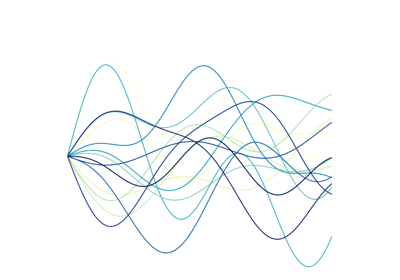# tslearn.preprocessing.TimeSeriesScalerMinMax¶

class tslearn.preprocessing.TimeSeriesScalerMinMax(value_range=(0.0, 1.0))[source]

Scaler for time series. Scales time series so that their span in each dimension is between min and max where value_range=(min, max).

Parameters: value_range : tuple (default: (0., 1.)) The minimum and maximum value for the output time series.

Notes

This method requires a dataset of equal-sized time series.

NaNs within a time series are ignored when calculating min and max.

Examples

>>> TimeSeriesScalerMinMax(value_range=(1., 2.)).fit_transform([[0, 3, 6]])
array([[[1. ],
[1.5],
[2. ]]])
>>> TimeSeriesScalerMinMax(value_range=(1., 2.)).fit_transform(
...     [[numpy.nan, 3, 6]]
... )
array([[[nan],
[ 1.],
[ 2.]]])


Methods

 fit(X[, y]) A dummy method such that it complies to the sklearn requirements. fit_transform(X[, y]) Fit to data, then transform it. get_params([deep]) Get parameters for this estimator. set_params(**params) Set the parameters of this estimator. transform(X[, y]) Will normalize (min-max) each of the timeseries.
fit(X, y=None, **kwargs)[source]

A dummy method such that it complies to the sklearn requirements. Since this method is completely stateless, it just returns itself.

Parameters: X Ignored self
fit_transform(X, y=None, **kwargs)[source]

Fit to data, then transform it.

Parameters: X : array-like of shape (n_ts, sz, d) Time series dataset to be rescaled. numpy.ndarray Resampled time series dataset.
get_params(deep=True)[source]

Get parameters for this estimator.

Parameters: deep : bool, default=True If True, will return the parameters for this estimator and contained subobjects that are estimators. params : dict Parameter names mapped to their values.
set_params(**params)[source]

Set the parameters of this estimator.

The method works on simple estimators as well as on nested objects (such as Pipeline). The latter have parameters of the form <component>__<parameter> so that it’s possible to update each component of a nested object.

Parameters: **params : dict Estimator parameters. self : estimator instance Estimator instance.
transform(X, y=None, **kwargs)[source]

Will normalize (min-max) each of the timeseries. IMPORTANT: this transformation is completely stateless, and is applied to each of the timeseries individually.

Parameters: X : array-like of shape (n_ts, sz, d) Time series dataset to be rescaled. numpy.ndarray Rescaled time series dataset.

## Examples using tslearn.preprocessing.TimeSeriesScalerMinMax¶Nearest neighborsHyper-parameter tuning of a Pipeline with KNeighborsTimeSeriesClassifierSoft-DTW weighted barycentersSVM and GAKLearning ShapeletsAligning discovered shapelets with timeseriesLearning Shapelets: decision boundaries in 2D distance space